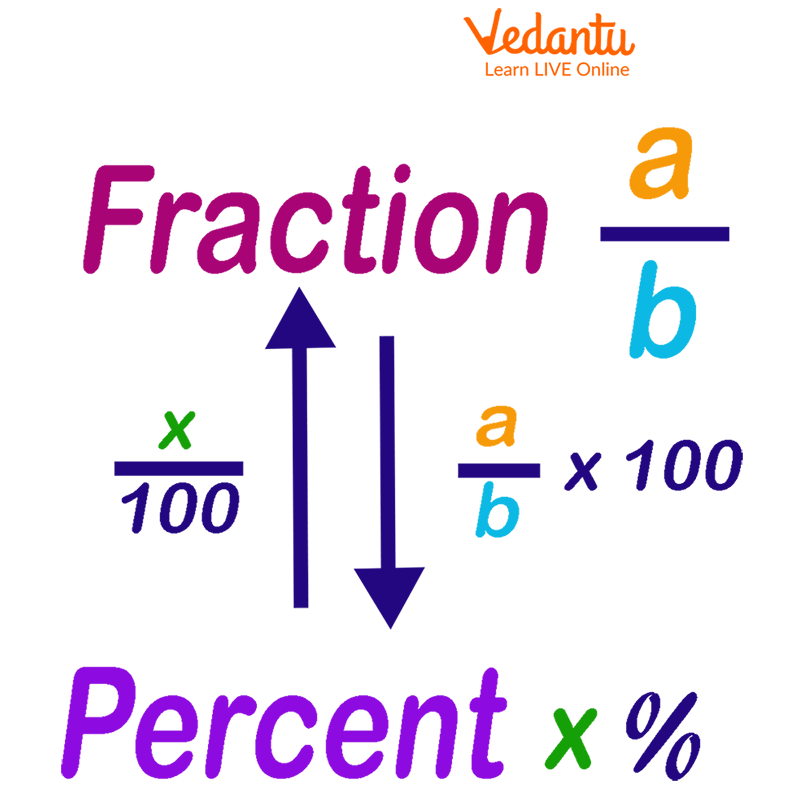Courses
Courses for Kids
Free study material
Free LIVE classes
More

# Fraction Value of 30%LIVE
Join Vedantu’s FREE Mastercalss

## 30 Percent as a Fraction in the Simplest Form

Suppose you have 10 candies and your brother takes 3 of your candies? Now, do you know what fraction of candies did he take? Well, he took $\frac{3}{10}$ of your candies. Let us learn what percentage of candies your brother took or what is the percentage value of $\frac{3}{10}$?

In the following sections, you will learn how to write any fraction into a respective percentage and vice-versa. Also, you will learn how to write $\frac{3}{10}$ in percentage here.

## What is a Fraction?

Fraction is any number that is written in the form of $\frac{a}{b}$. In the above fraction $\frac{3}{10}$, a = 3 is called the numerator and b = 10 is called the denominator.

## What is Percent?

Percent is a number or value per hundred. It is denoted by the symbol %.

## How to Convert Percentage in Fraction?

The conversion of percentage to a fraction is very easy. It involves two steps that are following:

Step 1. Remove the percentage symbol and divide by 100.

Step 2: Reduce the fraction to its simplest form.

## What is Fraction Value of 30 %?

By following the above-given steps, we can convert the 30 percent into a fraction.

Step 1. 30 % = $\frac{30}{100}$

Step 2. Reduce the fraction $\frac{30}{100}$

=$\frac{30}{100}$ = $\frac{3}{10}$

So, the fraction value of 30 % is $\frac{3}{10}$.

## How to Write Fractional Value into Percentage?

It is the reverse process. It is also a two-step process. The following are the two steps.

Step 1: Multiply the given fraction by 100 and add the % sign with it.

Step 2: Simplify the fraction and write the answer in integer or decimal numbers.

## Convert $\frac{3}{10}$ to its Percentage Value

$\frac{3}{10}$ can be written in percentage as:

Step 1: $\frac{3}{10}$ x 100 %

Step 2: 3 ｘ 10 % = 30%

So, $\frac{3}{10}$ can be written as 30%.

Similarly, we can write any number or fraction in percentage.Conversion from Fraction to Percent and Percent to Fraction

## Let’s see Another Common Fraction and its Percentage Values!

Let us now find the percentage value of $\frac{1}{2}$.

$\frac{1}{2}$ can be written in percentage as:

Step 1: $\frac{1}{2}$ x 100 %

Step 2: 1 ｘ 50 % = 50%

So, $\frac{1}{2}$ can be written as 50%.

## Points to Remember!

If a number is in decimal form, then first we need to convert the number into a fraction and then follow the above-given steps to convert a fraction into a percentage value.

Example: Write the percentage value of 0.3.

Solution: 0.3 = $\frac{3}{10}$

$\frac{3}{10}$ can be written in percentage as:

$\frac{3}{10}$x 100 % = 30 %

## Tips for Parents

Mathematics is the most scoring subject, and kids get better at it with practice. We suggest parents to let their kids understand the applications of various mathematical concepts like fractions and percentages, etc. in their day to day lives to get a good hold of the subject. For example, they can introduce the concept of fractions by sharing a slice of pizza and make learning a fun process.

## Conclusion

In this article, we have learnt the steps to convert any percentage value to its equivalent fraction and vice-versa. Also, we have converted the percentage value of 30 as a fraction.

Last updated date: 28th Sep 2023
Total views: 132.6k
Views today: 2.32k

## FAQs on Fraction Value of 30%

1. What is the percentage?

In Mathematics, the percentage is the rate, number, or amount in each hundred. It is denoted by the symbol ‘%’.

It also shows the quantity of any number or proportion with respect to the whole.

For example: 8% of votes are invalid. Here, 8 % is the share that represents the value with respect to the total number of votes.

2. What is the process to convert a fraction to its equivalent percentage value?

Let us consider a fraction $\frac{a}{b}$. To convert a fraction to its equivalent percentage value, we will follow two steps:

Step 1: Multiply 100 to the given fractional number and add the percent sign (%).

$\frac{a}{b}$ x 100%

Step 2: Simplify the expression.

(Put the value of a and b and solve the equation obtained in Step 1.)